# k map 2

We previously showed on the above right diagram that the white open region is (A’+B’)’. On an earlier example we showed a doubly hatched region at

the intersection (overlay) of AB. This is the left and middle figures repeated here. Comparing the two Venn diagrams, we see that this open region ,

(A’+B’)’, is the same as the doubly hatched region AB (A AND B). We can also prove that (A’+B’)’=AB by DeMorgan’s theorem and double

negation as shown above.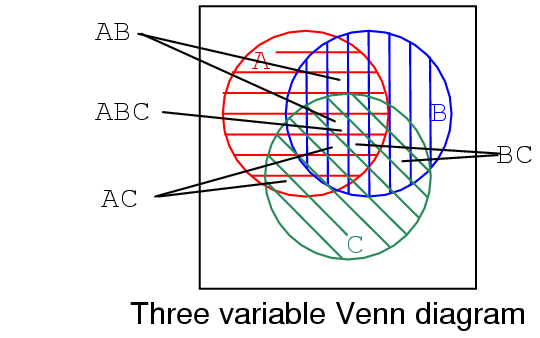We show a three variable Venn diagram above with regions A (red horizontal), B (blue vertical), and, C (green 45o). In the

very center note that all three regions overlap representing Boolean expression ABC. There is also a larger petal shaped region where A and

B overlap corresponding to Boolean expression AB. In a similar manner A and C overlap producing Boolean expression AC.

And B and C overlap producing Boolean expression BC.

Looking at the size of regios described by AND expressions above, we see that region size varies with the number of variables in the associated AND

expression.

A, 1-variable is a large circular region.
AB, 2-variable is a smaller petal shaped region.
ABC, 3-variable is the smallest region.
The more variables in the AND term, the smaller the region.

## Making a Venn diagram look like a Karnaugh map

Starting with circle A in a rectangular A’ universe in figure (a) below, we morph a Venn diagram into almost a Karnaugh map.We expand circle A at (b) and (c), conform to the rectangular A’ universe at (d), and change A to a rectangle at (e). Anything left

outside of A is A’ . We assign a rectangle to A’ at (f). Also, we do not use shading in Karnaugh maps. What we have so far

resembles a 1-variable Karnaugh map, but is of little utility. We need multiple variables.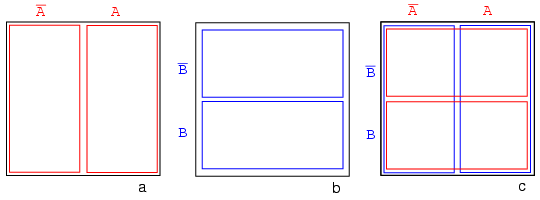Figure (a) above is the same as the previous Venn diagram showing A and A’ above except that the labels A and A’ are above the

diagram instead of inside the respective regions. Imagine that we have go through a process similar to figures (a-f) to get a “square Venn diagram” for

B and B’ as we show in middle figure (b). We will now superimpose the diagrams in Figures (a) and (b) to get the result at (c), just like we

have been doing for Venn diagrams. The reason we do this is so that we may observe that which may be common to two overlapping regions– say where A

overlaps B. The lower right cell in figure (c) corresponds to AB where A overlaps B.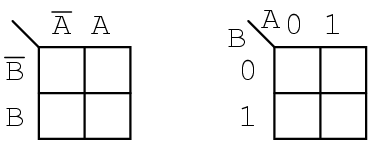We don’t waste time drawing a Karnaugh map like (c) above, sketching a simplified version as above left instead. The column of two cells under A’ is

understood to be associated with A’, and the heading A is associated with the column of cells under it. The row headed by B’ is

associated with the cells to the right of it. In a similar manner B is associated with the cells to the right of it. For the sake of simplicity, we

do not delineate the various regions as clearly as with Venn diagrams.

The Karnaugh map above right is an alternate form used in most texts. The names of the variables are listed next to the diagonal line. The A above

the diagonal indicates that the variable A (and A’) is assigned to the columns. The 0 is a substitute for A’, and the 1

substitutes for A. The B below the diagonal is associated with the rows: 0 for B’, and 1 for B

Example:

Mark the cell corresponding to the Boolean expression AB in the Karnaugh map above with a 1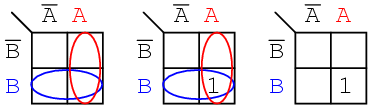Solution:

Shade or circle the region corresponding to A. Then, shade or enclose the region corresponding to B. The overlap of the two regions is

AB. Place a 1 in this cell. We do not necessarily enclose the A and B regions as at above left.We develop a 3-variable Karnaugh map above, starting with Venn diagram like regions. The universe (inside the black rectangle) is split into two narrow

narrow rectangular regions for A’ and A. The variables B’ and B divide the universe into two square regions. C occupies a

square region in the middle of the rectangle, with C’ split into two vertical rectangles on each side of the C square.

In the final figure, we superimposeall three variables, attempting to clearly label the various regions. The regions are less obvious without color

printing, more obvious when compared to the other three figures. This 3-variable K-Map (Karnaugh map) has 23 = 8 cells, the small

squares within the map. Each individual cell is uniquely identified by the three
Boolean Variables (A, B, C). For example, ABC’ uniquely selects the lower right most cell(*), A’B’C’ selects the upper left most cell

(x).We don’t normally label the Karnaugh map as shown above left. Though this figure clearly shows map coverage by single boolean variables of a 4-cell region.

Karnaugh maps are labeled like the illustration at right. Each cell is still uniquely identified by a 3-variable product term, a Boolean AND

expression. Take, for example, ABC’ following the A row across to the right and the BC’ column down, both intersecting at the lower

right cell ABC’. See (*) above figure.The above two different forms of a 3-variable Karnaugh map are equivalent, and is the final form that it takes. The version at right is a bit easier to use,

since we do not have to write down so many boolean alphabetic headers and complement bars, just 1s and 0s Use the form of map on the right and

look for the the one at left in some texts. The column headers on the left B’C’, B’C, BC, BC’ are equivalent to 00, 01, 11, 10 on the right.

The row headers A, A’ are equivalent to 0, 1 on the right map.

## Karnaugh maps, truth tables, and Boolean expressions

Maurice Karnaugh, a telecommunications engineer, developed the Karnaugh map at Bell Labs in 1953 while designing digital logic based telephone switching

circuits.

Now that we have developed the Karnaugh map with the aid of Venn diagrams, let’s put it to use. Karnaugh maps reduce logic functions more quickly and

easily compared to Boolean algebra. By reduce we mean simplify, reducing the number of gates and inputs. We like to simplify logic to a lowest cost

form to save costs by elimination of components. We define lowest cost as being the lowest number of gates with the lowest number of inputs per gate.

Given a choice, most students do logic simplification with Karnaugh maps rather than Boolean algebra once they learn this tool.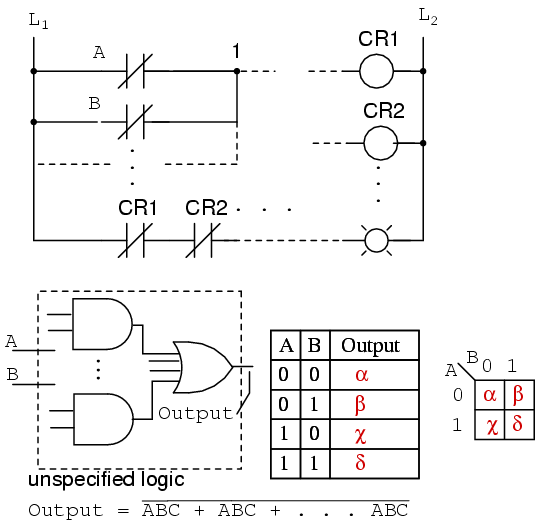We show five individual items above, which are just different ways of representing the same thing: an arbitrary 2-input digital logic function. First is

relay ladder logic, then logic gates, a truth table, a Karnaugh map, and a Boolean equation. The point is that any of these are equivalent. Two inputs

A and B can take on values of either 0 or 1, high or low, open or closed, True or False, as the case may be. There are 22

= 4 combinations of inputs producing an output. This is applicable to all five examples.

These four outputs may be observed on a lamp in the relay ladder logic, on a logic probe on the gate diagram. These outputs may be recorded in the truth

table, or in the Karnaugh map. Look at the Karnaugh map as being a rearranged truth table. The Output of the Boolean equation may be computed by the laws

of Boolean algebra and transfered to the truth table or Karnaugh map. Which of the five equivalent logic descriptions should we use? The one which is most

useful for the task to be accomplished.The outputs of a truth table correspond on a one-to-one basis to Karnaugh map entries. Starting at the top of the truth table, the A=0, B=0 inputs

produce an output &#94;. Note that this same output α is found in the Karnaugh map at the A=0, B=0 cell address, upper left corner of K-map where the

A=0 row and B=0 column intersect. The other truth table outputs β, χ, δ from inputs AB=01, 10, 11 are found at corresponding K-map

locations.

Below, we show the adjacent 2-cell regions in the 2-variable K-map with the aid of previous rectangular Venn diagram like Boolean regions.Cells α and χ are adjacent in the K-map as ellipses in the left most K-map below. Referring to the previous truth table, this is not the case.

There is another truth table entry (β) between them. Which brings us to the whole point of the organizing the K-map into a square array, cells with any

Boolean variables in common need to be close to one another so as to present a pattern that jumps out at us. For cells α and χ they have the

Boolean variable B’ in common. We know this because B=0 (same as B’) for the column above cells α and χ. Compare this to the

square Venn diagram above the K-map.

A similar line of reasoning shows that β and δ have Boolean B (B=1) in common. Then, α and β have Boolean A’ (A=0) in

common.
Finally, χ and δ have Boolean A (A=1) in common. Compare the last two maps to the middle square Venn diagram.

To summarize, we are looking for commonality of Boolean variables among cells. The Karnaugh map is organized so that we may see that commonality. Let’s try

some examples.Example:

Transfer the contents of the truth table to the Karnaugh map above.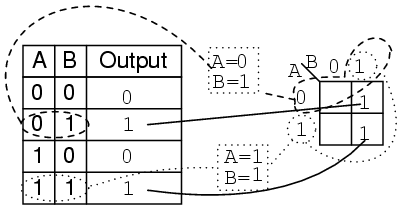Solution:

The truth table contains two 1s. the K- map must have both of them. locate the first 1 in the 2nd row of the truth table above.

note the truth table AB address
locate the cell in the K-map having the same address
place a 1 in that cell

Repeat the process for the 1 in the last line of the truth table.

Example:

For the Karnaugh map in the above problem, write the Boolean expression. Solution is below.Solution:

Look for adjacent cells, that is, above or to the side of a cell. Diagonal cells are not adjacent. Adjacent cells will have one or more Boolean variables in

common.

Group (circle) the two 1s in the column
Find the variable(s) top and/or side which are the same for the group, Write this as the Boolean result. It is B in our case.
Ignore variable(s) which are not the same for a cell group. In our case A varies, is both 1 and 0, ignore Boolean A.
Ignore any variable not associated with cells containing 1s. B’ has no ones under it. Ignore B’
Result Out = B

This might be easier to see by comparing to the Venn diagrams to the right, specifically the B column.

Example:

Write the Boolean expression for the Karnaugh map below.Solution: (above)

Group (circle) the two 1’s in the row
Find the variable(s) which are the same for the group, Out = A’

Example:

For the Truth table below, transfer the outputs to the Karnaugh, then write the Boolean expression for the result.Solution:

Transfer the 1s from the locations in the Truth table to the corresponding locations in the K-map.

Group (crcle) the two 1’s in the column under B=1
Group (circle) the two 1’s in the row right of A=1
Write product term for first group = B
Write product term for second group = A
Write Sum-Of-Products of above two terms Output = A+B

The solution of the K-map in the middle is the simplest or lowest cost solution. A less desirable solution is at far right. After grouping the two

1s, we make the mistake of forming a group of 1-cell. The reason that this is not desirable is that:

The single cell has a product term of AB’
The corresponding solution is Output = AB’ + B
This is not the simplest solution

The way to pick up this single 1 is to form a group of two with the 1 to the right of it as shown in the lower line of the middle K-map, even

though this 1 has already been included in the column group (B). We are allowed to re-use cells in order to form larger groups. In fact, it is

desirable because it leads to a simpler result.

We need to point out that either of the above solutions, Output or Wrong Output, are logically correct. Both circuits yield the same output. It is a matter

of the former circuit being the lowest cost solution.

Example:

Fill in the Karnaugh map for the Boolean expression below, then write the Boolean expression for the result.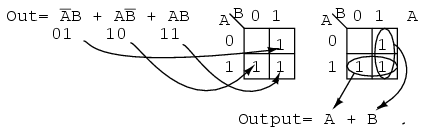Solution: (above)

The Boolean expression has three product terms. There will be a 1 entered for each product term. Though, in general, the number of 1s per

product term varies with the number of variables in the product term compared to the size of the K-map. The product term is the address of the cell where

the 1 is entered. The first product term, A’B, corresponds to the 01 cell in the map. A 1 is entered in this cell. The other

two P-terms are entered for a total of three 1s

Next, proceed with grouping and extracting the simplified result as in the previous truth table problem.

Example:

Simplify the logic diagram below.Solution: (Figure below)

Write the Boolean expression for the original logic diagram as shown below
Transfer the product terms to the Karnaugh map
Form groups of cells as in previous examples
Write Boolean expression for groups as in previous examples
Draw simplified logic diagramExample:

Simplify the logic diagram below.Solution:

Write the Boolean expression for the original logic diagram shown above
Transfer the product terms to the Karnaugh map.
It is not possible to form groups.
No simplification is possible; leave it as it is.

No logic simplification is possible for the above diagram. This sometimes happens. Neither the methods of Karnaugh maps nor Boolean algebra can simplify

this logic further. We show an Exclusive-OR schematic symbol above; however, this is not a logical simplification. It just makes a schematic diagram look

nicer. Since it is not possible to simplify the Exclusive-OR logic and it is widely used, it is provided by manufacturers as a basic integrated circuit

(7486).

## Logic simplification with Karnaugh maps

The logic simplification examples that we have done so could have been performed with Boolean algebra about as quickly. Real world logic simplification

problems call for larger Karnaugh maps so that we may do serious work. We will work some contrived examples in this section, leaving most of the real world

applications for the Combinatorial Logic chapter. By contrived, we man examples which illustrate techniques. This approach will develop the tools we need

to transition to the more complex applications in the Combinatorial Logic chapter.

We show our previously developed Karnaugh map. We will use the form on the rightNote the sequence of numbers across the top of the map. It is not in binary sequence which would be 00, 01, 10, 11. It is 00, 01, 11 10, which

is Gray code sequence. Gray code sequence only changes one binary bit as we go from one number to the next in the sequence, unlike binary. That means that

adjacent cells will only vary by one bit, or Boolean variable. This is what we need to organize the outputs of a logic function so that we may view

commonality. Moreover, the column and row headings must be in Gray code order, or the map will not work as a Karnaugh map. Cells sharing common Boolean

variables would no longer be adjacent, nor show visual patterns. Adjacent cells vary by only one bit because a Gray code sequence varies by only one bit.

If we sketch our own Karnaugh maps, we need to generate Gray code for any size map that we may use. This is how we generate Gray code of any size.Note that the Gray code sequence, above right, only varies by one bit as we go down the list, or bottom to top up the list. This property of Gray code is

often useful in digital electronics in general. In particular, it is applicable to Karnaugh maps.

Let us move on to some examples of simplification with 3-variable Karnaugh maps. We show how to map the product terms of the unsimplified logic to the

K-map. We illustrate how to identify groups of adjacent cells which leads to a Sum-of-Products simplification of the digital logic.Above we, place the 1’s in the K-map for each of the product terms, identify a group of two, then write a p-term (product term) for the sole group

as our simplified result.Mapping the four product terms above yields a group of four covered by Boolean A’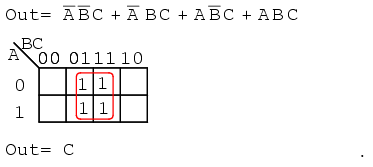Mapping the four p-terms yields a group of four, which is covered by one variable C.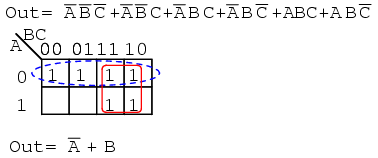After mapping the six p-terms above, identify the upper group of four, pick up the lower two cells as a group of four by sharing the two with two more from

the other group. Covering these two with a group of four gives a simpler result. Since there are two groups, there will be two p-terms in the

Sum-of-Products result A’+B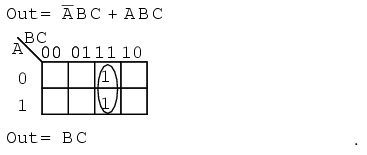The two product terms above form one group of two and simplifies to BC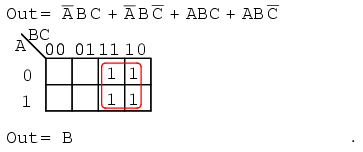Mapping the four p-terms yields a single group of four, which is BMapping the four p-terms above yields a group of four. Visualize the group of four by rolling up the ends of the map to form a cylinder, then the cells are

adjacent. We normally mark the group of four as above left. Out of the variables A, B, C, there is a common variable: C’. C’ is a 0 over all four cells.

Final result is C’.The six cells above from the unsimplified equation can be organized into two groups of four. These two groups should give us two p-terms in our simplified

result of A’ + C’.

Below, we revisit the Toxic Waste Incinerator from the Boolean algebra hapter. See Boolean algebra chapter for details on this example. We will simplify

the logic using a Karnaugh map.The Boolean equation for the output has four product terms. Map four 1’s corresponding to the p-terms. Forming groups of cells, we have three groups of two.

There will be three p-terms in the simplified result, one for each group. See “Toxic Waste Incinerator”, Boolean algebra chapter for a gate diagram of the

result, which is reproduced below.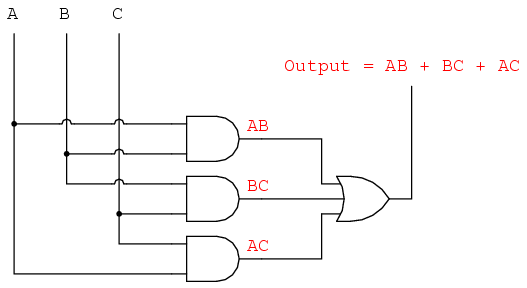Below we repeat the Boolean algebra simplification of Toxic waste incinerator for comparison.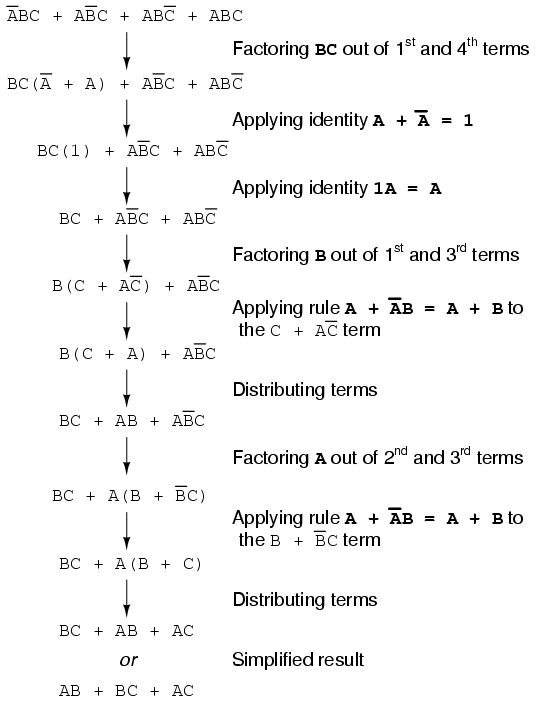Below we repeat the Toxic waste incinerator Karnaugh map solution for comparison to the above Boolean algebra simplification. This case illustrates why the

Karnaugh map is widely used for logic simplification.The Karnaugh map method looks easier than the previous page of
boolean algebra.

Related

COMMENT songs